# Separately continuous not implies continuous

This article gives the statement and possibly, proof, of a non-implication relation between two function properties. That is, it states that every function satisfying the first function property (i.e., separately continuous function) need not satisfy the second function property (i.e., continuous function)
View a complete list of function property non-implications | View a complete list of function property implications
Get more facts about separately continuous function|Get more facts about continuous function
EXPLORE EXAMPLES YOURSELF: View examples of subgroups satisfying property separately continuous function but not continuous function|View examples of subgroups satisfying property separately continuous function and continuous function

## Statement

### For a function of two variables at a point

It is possible to have the following: a function$f$ of two variables$x,y$ and a point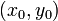$(x_0,y_0)$ in the domain of the function such that$f$ is separately continuous in both variables$x,y$ at the point$(x_0,y_0)$, but is not a continuous function at$(x_0,y_0)$.

### For a function of two variables overall

It is possible to have the following: a function$f$ of two variables$x,y$ that is separately continuous in both variables$x,y$ everywhere on$\R^2$, but is not continuous everywhere on$\R^2$ i.e., there is some point in$\R^2$ where$f$ is not continuous.

### For a function of multiple variables

We can replace functions of two variables by functions of more than two variables in both the above formulations.

## Proof

### Example

We give a single example that illustrates both versions of the statement.

Consider the function: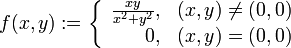$f(x,y) := \left\lbrace \begin{array}{rl} \frac{xy}{x^2 + y^2}, & (x,y) \ne (0,0) \\ 0, & (x,y) = (0,0) \\\end{array}\right.$

It's clear that$f$ is continuous, as well as separately continuous, at all points other than$(0,0)$. At the point$(0,0)$, we calculate the limits along the$x$-direction and$y$-direction:

• The limit along the$x$-direction is$\lim_{x \to 0} \frac{(x)(0)}{x^2 + 0^2} = \lim_{x \to 0} 0 = 0$. This coincides with the value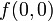$f(0,0)$.
• The limit along the$y$-direction is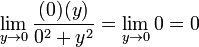$\lim_{y \to 0} \frac{(0)(y)}{0^2 + y^2} = \lim_{y \to 0} 0 = 0$. This coincides with the value$f(0,0)$.

Thus, the function$f$ is separately continuous in both$x$ and$y$ at the point$(0,0)$. Since we already established that it's separately continuous everywhere else, we obtain that it is separately continuous on all of$\R^2$.

On the other hand,$f$ is not continuous at$(0,0)$. To see this, consider the limit along the line$y = x$. Setting$y = x$, we get that the limit is:$\lim_{x \to 0} \frac{xx}{x^2 + x^2} = \lim_{x \to 0} \frac{x^2}{2x^2} = \lim_{x \to 0}\frac{1}{2} = \frac{1}{2} \ne f(0,0)$

Note that if the function were indeed continuous at$(0,0)$, the limit along every direction would equal the value at the point, so this shows that the function is not continuous at$(0,0)$.

### Idea behind example

The secret behind the example can be better understood using polar coordinates, though this is not necessary to understand the example itself. In polar coordinates, we can rewrite$f(x,y) = g(r,\theta)$ where:$g(r,\theta) = \left \lbrace \begin{array}{rl} \frac{1}{2}\sin(2\theta), & r \ne 0\\ 0, & r = 0 \\\end{array}\right.$

Here's how we get this. Plug in$x = r \cos \theta$ and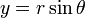$y = r \sin \theta$ in the original expression for points other than the origin. We have: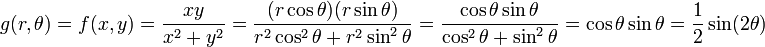$g(r,\theta) = f(x,y) = \frac{xy}{x^2 + y^2} = \frac{(r\cos \theta)(r \sin \theta)}{r^2 \cos^2\theta + r^2 \sin^2\theta} = \frac{\cos \theta \sin \theta}{\cos^2\theta + \sin^2\theta} = \cos \theta \sin \theta = \frac{1}{2}\sin(2\theta)$

The limit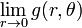$\lim_{r \to 0} g(r,\theta)$ for any fixed value of$\theta$, is the number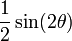$\frac{1}{2}\sin(2\theta)$. Thus:

• If$\theta$ is a multiple of$\pi/2$ (this includes the positive$x$-direction, positive$y$-direction, negative$x$-direction, and negative$y$-direction), the limit at the origin along the half-line corresponding to$\theta$ is zero. This explains why the function is continuous along the$x$- and$y$-directions, hence separately continuous in the Cartesian coordinate system.
• If$\theta$ is not a multiple of$\pi/2$, the limit at the origin along the half-line corresponding to$\theta$ is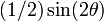$(1/2)\sin(2\theta)$, a nonzero number. This limit does not equal the value, hence the function is not continuous in this direction. Hence, the function is not continuous.

### Extending the example

We can generalize the idea behind the example to obtain new examples of functions that are continuous from many directions but still not continuous as functions. For instance, the function that in polar coordinates would read as:$\left \lbrace \begin{array}{rl} \sin(4\theta) & r \ne 0 \\ 0, & r = 0 \\\end{array}\right.$

is continuous not just along the$x$- and$y$-directions but also along the$y = x$ and$y = -x$ lines. However, it is still not continuous from every direction, hence is not a continuous function.

It is even possible to construct examples of functions that are continuous in every linear direction but not continuous as functions. See continuous in every linear direction not implies continuous.

### Generalization to functions of more than two variables

We can use the same example as for a function of two variables, with the above functional form in terms of two of the input variables, and with the function independent of the remaining variables.